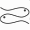# Threeleaved Rose

This model is the level set of f(x,y) = (x^2 + y^2)^2 - 4 a y x^2 + a y (x^2+y^2)

The singularity at the origin for this function has six tangent directions. The function is extremely flat near the singularity, so this model is a good test-case for singularity-detection.

This function can also be defined in the polar form as r= a sin(3 theta)

Previous: ModelsThe Pisces Home Page